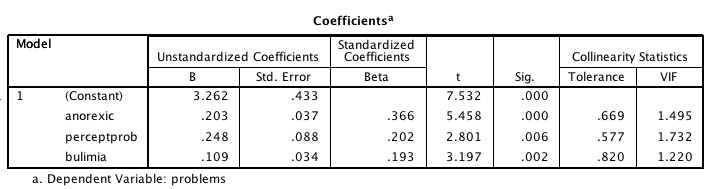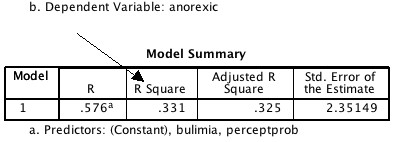# Multicollinearity statistics with SPSS

“Can you explain multicollinearity statistics?”

Why, yes, yes I can.

First of all, as noted in the Journal of Polymorphous Perversity,

“Multicollinearity is not a life-threatening condition except when a depressed graduate student employs multiple, redundant measures.”

What is multicollinearity, then, and how do you know if you have it?

Multicollinearity is a problem that occurs with regression analysis when there is a high correlation of at least one independent variable with a combination of the other independent variables. The most extreme example of this would be if you did something like had two completely overlapping variables. Say you were predicting income from the Excellent Test for Income Prediction (ETIP). Unfortunately, you are a better test designer than statistician so your two independent variables are Number of Answers Correct (CORRECT)  and Number of Answers Incorrect (INCORRECT). Those two are going to have a perfect negative correlation of -1.  Multicollinearity. You are not going to be able to find a single least squares solution. For example, if you have this equation:

Income = .5*Correct + 0*Incorrect

or

Income = 0*Correct -.5*Incorrect

You will get the exact same prediction.  Now that is a pretty trivial example, but you can have a similar problem if you use two or more predictors that are very highly correlated. Let’s assume you’re predicting income from high school GPA, college GPA and SAT score. It may be that high school GPA and SAT score together have a very high multiple correlation with college GPA.For more about why multicollinearity is a bad thing, read this very nice web page by a person in Michigan who I don’t know. Let’s say you already know multicollinearity is bad and you want to know how to spot it, kind of like cheating boyfriends. Well, I can’t help you with THAT (although you can try looking for lipstick on his collar), but I can help you with multicollinearity.

One suggestion some people give is to look at your correlation matrix and see if you have any independent variables that correlate above some level with one another. Some people say .75, some say .90, some say potato. I say that looking at your correlation matrix is fine as far as it goes, but it doesn’t go far enough. Certainly if I had variables correlated above .90 I would not include both in the equation. Even if it was above .75, I would look a bit askance, but I might go ahead and try it anyway and see the results.The problem with just looking at the correlation matrix is what if you have four variables that together explain 100% of the variance in a fifth independent variable. You aren’t going to be able to tell that by just looking at the correlation matrix. Enter the Tolerance Statistic, wearing a black cape, here to save the day. Okay, I lied, it isn’t really wearing a black cape  – it’s a green cape. ( By the way, if you have a mad urge to buy said green cape, or a Viking tunic, you can fulfill your desires here. I am not affiliated with this website in any way. I am just impressed that they seem to be finding a niche in the Pirate Garb / Viking tunic / cloak market .)

In complete seriousness now, ahem ….

To compute a tolerance statistic for an independent variable to test for multi-collinearity, a multiple regression is performed with that variable as the new dependent and all of the other independent variables in the model as independent variables. The tolerance statistic is 1 – R2 for this second  regression. (R-square, just to remind you, is the amount of variance in a dependent variable in a multiple regression explained by a combination of all of the indepedent  variables). In other words, Tolerance is 1 minus the amount of variance in the independent variable explained by all of the other independent variables. A tolerance statistic below .20 is generally considered cause for concern.Of course, in real life, you don’t actually compute a bunch of regressions with all of your independent variables as dependents, you just look at the collinearity statistics.

Let’s take a look at an example in SPSS, shall we?

The code is below or you can just pick REGRESSION from the ANALYZE menu. Don’t forget to click on the STATISTICS button and select COLLINEARITY STATISTICS.

Here I have a dependent variable that is the rating of problems a person has with sexual behavior, sexual attitudes and mental state. The three independent variables are ratings of symptoms of anorexia, symptoms of bulimia and problems in body perception

REGRESSION
/MISSING LISTWISE
/STATISTICS COEFF OUTS R ANOVA COLLIN TOL
/CRITERIA=PIN(.05) POUT(.10)
/NOORIGIN
/DEPENDENT problems
/METHOD=ENTER anorexic perceptprob bulimia.Let’s just take a look at the first variable “anorexic”. It has a Tolerance of .669.  What does that mean? It means that if I ran a multiple regression with anorexic as the dependent, and perceptprob and bulimia as the independent vairables, I would get an R-square value of .331. Don’t take my word for it. Let’s try it. Notice that now anorexic is the dependent variable.

REGRESSION
/MISSING LISTWISE
/STATISTICS COEFF OUTS R ANOVA COLLIN TOL
/CRITERIA=PIN(.05) POUT(.10)
/NOORIGIN
/DEPENDENT anorexic
/METHOD=ENTER perceptprob bulimia.Now, look at that. When we do a regression with anorexia as the dependent variable and bulimia and perceptprob as the two independent variables the R-square is .331 . If we take 1 – .331 we get .669 which is exactly the Tolerance Statistic for anorexia in the previous regression analysis above. Don’t you just love it when everything works out?

So WHY is a tolerance below .20 considered a cause for concern? It means that at least 80% of the variance of this independent variable is shared with some other independent variables. It means that the multiple correlation of the other independent variables with this independent variable is at least .90 (because .9 * .9 = .81) .

Another statistic sometimes used for multicollinearity is the Variance Inflation Factor, which is just the reciprocal of the tolerance statistics. A VIF of greater than 5 is generally considered evidence of multicollinearity. If you divide 1 by .669 you’ll get 1.495, which is exactly the same as the VIF statistic shown above .

And you thought I just made this sh*t up as I went along, didn’t you?

Have kids? Know anyone who has kids? Like kids? Own a computer? Fish Lake will teach fractions and Native American history, with no whining and all for under ten bucks.## Similar Posts

1.Lyla says: